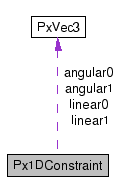# Px1DConstraint Struct Reference [Physics]

A constraint. More...

`#include <PxConstraintDesc.h>`

Collaboration diagram for Px1DConstraint:[legend]

## Public Attributes

PxVec3 linear0
linear component of velocity jacobian in world space
PxReal geometricError
geometric error of the constraint along this axis
PxVec3 angular0
angular component of velocity jacobian in world space
PxReal velocityTarget
velocity target for the constraint along this axis
PxVec3 linear1
linear component of velocity jacobian in world space
PxReal minImpulse
minimum impulse the solver may apply to enforce this constraint
PxVec3 angular1
angular component of velocity jacobian in world space
PxReal maxImpulse
maximum impulse the solver may apply to enforce this constraint
union {
struct SpringModifiers {
PxReal   stiffness
spring parameter, for spring constraints
PxReal   damping
damping parameter, for spring constraints
}   spring
struct RestitutionModifiers {
PxReal   restitution
restitution parameter for determining additional "bounce"
PxReal   velocityThreshold
minimum impact velocity for bounce
}   bounce
mods
PxReal forInternalUse
for internal use only
PxU16 flags
a set of Px1DConstraintFlags
PxU16 solveHint
constraint optimization hint, should be an element of PxConstraintSolveHint

## Detailed Description

A constraint.

A constraint is expressed as a set of 1-dimensional constraint rows which define the required constraint on the objects' velocities.

Each constraint is either a hard constraint or a spring. We define the velocity at the constraint to be the quantity

v = body0vel.dot(lin0,ang0) - body1vel.dot(lin1, ang1)

For a hard constraint, the solver attempts to generate

1. a set of velocities for the objects which, when integrated, respect the constraint errors:

v + (geometricError / timestep) = velocityTarget

2. a set of velocities for the objects which respect the constraints:

v = velocityTarget

Hard constraints support restitution: if the impact velocity exceeds the bounce threshold, then the target velocity of the constraint will be set to restitution * -v

Alternatively, the solver can attempt to resolve the velocity constraint as an implicit spring:

F = stiffness * -geometricError + damping * (velocityTarget - v)

where F is the constraint force or acceleration. Springs are fully implicit: that is, the force or acceleration is a function of the position and velocity after the solve.

All constraints support limits on the minimum or maximum impulse applied.

## Member Data Documentation

angular component of velocity jacobian in world space

angular component of velocity jacobian in world space

 struct { ... } ::RestitutionModifiers Px1DConstraint::bounce

 PxReal Px1DConstraint::damping

damping parameter, for spring constraints

 PxU16 Px1DConstraint::flags

a set of Px1DConstraintFlags

for internal use only

geometric error of the constraint along this axis

linear component of velocity jacobian in world space

linear component of velocity jacobian in world space

 PxReal Px1DConstraint::maxImpulse

maximum impulse the solver may apply to enforce this constraint

 PxReal Px1DConstraint::minImpulse

minimum impulse the solver may apply to enforce this constraint

 union { ... } Px1DConstraint::mods

 PxReal Px1DConstraint::restitution

restitution parameter for determining additional "bounce"

constraint optimization hint, should be an element of PxConstraintSolveHint

 struct { ... } ::SpringModifiers Px1DConstraint::spring

 PxReal Px1DConstraint::stiffness

spring parameter, for spring constraints

velocity target for the constraint along this axis

minimum impact velocity for bounce

The documentation for this struct was generated from the following file: